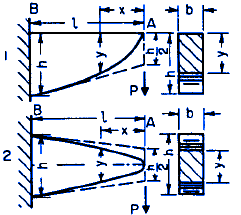Variable Shape Fixed End Single Concentrated Force Applied

Beam Deflection Equation Calculator with Variable Shape Fixed End Single Concentrated Force Applied

• Cross Section: Rectangle width b constant and depth y variable.
• Elevation 1) Top straight line and bottom patabola. 2) Complete parabola.
• Plan: rectangleALL calculators require a Premium Membership

Equations

Ss = I / c2

c2 = (5 b) / 8

I2 = (19 b3 d) / 480

y2 = (6 P x) / (b Ss )

h = (6 P l / b Ss)1/2

f = ( 8 P / b E ) ( l / h )3

Where:

 E = Modulus of Elasticity psi (N/mm2) I = Moment of Inertia in4 (mm4) P = Force lbf (N) h = Deflection inches (mm) Se= Section Modulus a, b, c, d, x, l = Some distance as indicated inches (mm)

References:

• Civil Engineering Formulas, Tyler G. Hicks, PE International Engineering Associates© Copyright 2000 - 2019, by Engineers Edge, LLC www.engineersedge.comMembership Register | LoginHomeEngineering Book StoreEngineering ForumExcel App. DownloadsOnline Books & ManualsEngineering NewsEngineering VideosEngineering CalculatorsEngineering ToolboxGD&T Training Geometric Dimensioning TolerancingDFM DFA TrainingTraining Online EngineeringAdvertising CenterCopyright Notice